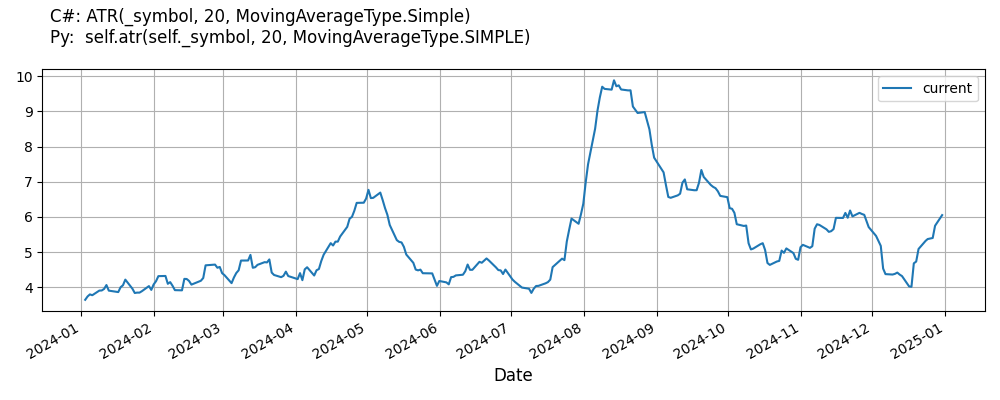# Supported Indicators

## Average True Range

### Introduction

The AverageTrueRange indicator is a measure of volatility introduced by Welles Wilder in his book: New Concepts in Technical Trading Systems. This indicator computes the TrueRange and then smoothes the TrueRange over a given period. TrueRange is defined as the maximum of the following: High - Low ABS(High - PreviousClose) ABS(Low - PreviousClose)

To view the implementation of this indicator, see the LEAN GitHub repository.

### Using ATR Indicator

To create an automatic indicators for AverageTrueRange, call the ATR helper method from the QCAlgorithm class. The ATR method creates a AverageTrueRange object, hooks it up for automatic updates, and returns it so you can used it in your algorithm. In most cases, you should call the helper method in the Initialize method.

public class AverageTrueRangeAlgorithm : QCAlgorithm
{
private Symbol _symbol;
private AverageTrueRange _atr;

public override void Initialize()
{
_atr = ATR("SPY", 20, MovingAverageType.Simple);
}

public override void OnData(Slice data)
{
{
// The current value of _atr is represented by itself (_atr)
// or _atr.Current.Value
Plot("AverageTrueRange", "atr", _atr);
// Plot all properties of atr
Plot("AverageTrueRange", "truerange", _atr.TrueRange);
}
}
}
class AverageTrueRangeAlgorithm(QCAlgorithm):
def Initialize(self) -> None:
self.atr = self.ATR("SPY", 20, MovingAverageType.Simple)

def OnData(self, slice: Slice) -> None:
# The current value of self.atr is represented by self.atr.Current.Value
self.Plot("AverageTrueRange", "atr", self.atr.Current.Value)
# Plot all attributes of self.atr
self.Plot("AverageTrueRange", "truerange", self.atr.TrueRange.Current.Value)


The following reference table describes the ATR method:

### ATR()1/1

            AverageTrueRange QuantConnect.Algorithm.QCAlgorithm.ATR (
Symbol                                symbol,
Int32                                 period,
*MovingAverageType                    type,
*Nullable<Resolution>           resolution,
*Func<IBaseData, IBaseDataBar>  selector
)


Creates a new AverageTrueRange indicator for the symbol. The indicator will be automatically updated on the given resolution.

If you don't provide a resolution, it defaults to the security resolution. If you provide a resolution, it must be greater than or equal to the resolution of the security. For instance, if you subscribe to hourly data for a security, you should update its indicator with data that spans 1 hour or longer.

The following table describes the MovingAverageType enumeration members:

You can manually create a AverageTrueRange indicator, so it doesn't automatically update. Manual indicators let you update their values with any data you choose.

Updating your indicator manually enables you to control when the indicator is updated and what data you use to update it. To manually update the indicator, call the Update method with a TradeBar, or QuoteBar. The indicator will only be ready after you prime it with enough data.

public class AverageTrueRangeAlgorithm : QCAlgorithm
{
private Symbol _symbol;
private AverageTrueRange _atr;

public override void Initialize()
{
_atr = new AverageTrueRange(20, MovingAverageType.Simple);
}

public override void OnData(Slice data)
{
if (data.Bars.TryGeValue(_symbol, out var bar))
{
_atr.Update(bar);
}

{
// The current value of _atr is represented by itself (_atr)
// or _atr.Current.Value
Plot("AverageTrueRange", "atr", _atr);
// Plot all properties of atr
Plot("AverageTrueRange", "truerange", _atr.TrueRange);
}
}
}
class AverageTrueRangeAlgorithm(QCAlgorithm):
def Initialize(self) -> None:
self.atr = AverageTrueRange(20, MovingAverageType.Simple)

def OnData(self, slice: Slice) -> None:
bar = slice.Bars.get(self.symbol)
if bar:
self.atr.Update(bar)

# The current value of self.atr is represented by self.atr.Current.Value
self.Plot("AverageTrueRange", "atr", self.atr.Current.Value)
# Plot all attributes of self.atr
self.Plot("AverageTrueRange", "truerange", self.atr.TrueRange.Current.Value)


To register a manual indicator for automatic updates with the security data, call the RegisterIndicator method.

public class AverageTrueRangeAlgorithm : QCAlgorithm
{
private Symbol _symbol;
private AverageTrueRange _atr;

public override void Initialize()
{
_atr = new AverageTrueRange(20, MovingAverageType.Simple);
RegisterIndicator(_symbol, _atr, Resolution.Daily);
}

public override void OnData(Slice data)
{
{
// The current value of _atr is represented by itself (_atr)
// or _atr.Current.Value
Plot("AverageTrueRange", "atr", _atr);
// Plot all properties of atr
Plot("AverageTrueRange", "truerange", _atr.TrueRange);
}
}
}
class AverageTrueRangeAlgorithm(QCAlgorithm):
def Initialize(self) -> None:
self.atr = AverageTrueRange(20, MovingAverageType.Simple)
self.RegisterIndicator(self.symbol, self.atr, Resolution.Daily)

def OnData(self, slice: Slice) -> None:
# The current value of self.atr is represented by self.atr.Current.Value
self.Plot("AverageTrueRange", "atr", self.atr.Current.Value)
# Plot all attributes of self.atr
self.Plot("AverageTrueRange", "truerange", self.atr.TrueRange.Current.Value)


The following reference table describes the AverageTrueRange constructor:

### AverageTrueRange()1/2

            AverageTrueRange QuantConnect.Indicators.AverageTrueRange (
string              name,
int                 period,
*MovingAverageType  movingAverageType
)


Creates a new AverageTrueRange indicator using the specified period and moving average type.

### AverageTrueRange()2/2

            AverageTrueRange QuantConnect.Indicators.AverageTrueRange (
int                 period,
*MovingAverageType  movingAverageType
)


Creates a new AverageTrueRange indicator using the specified period and moving average type.

### Visualization

The following image shows plot values of selected properties of AverageTrueRange using the plotly library.You can also see our Videos. You can also get in touch with us via Discord.